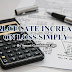The most important part in auto bet is to calculate how much to add on increase on loss. There is no fixed rule on this one.

But its recommend to not make it minimum that you will not earn at all or make it too high that you cannot survive an average loss streak. Set it by considering this properly.

So here is how to calculate increase on loss multiplier or percentage:

Some sites do not have the option to add increase on loss % and you have to add it in the form of a multiplier on loss. If you want to convert the increase on loss % to multiplier than use:

Multiplier on loss = 1+( Increase on loss % /100)

Example: For 35% increase on loss, the multiplier on loss is 1+(35/100) = 1+0.35 = 1.35

General formula:

This is the one simple formula that you can use for all payouts above 2.

➤Increase on loss = Winning Chance x 2 + House Edge.

So if you are playing on 50% Winning Chance and 1% House Edge.

Then, Increase on loss = 50 x 2 + 1 = 100+1 = 101

For Winning Chance below 1 % exclude the house edge.

➤Increase on loss for winning chance below 1% = Winning Chance x 2.

So for 0.25% Winning Chance increase on loss will be 0.25 x 2 = 0.5

This is for fast calculation purpose. Increase on loss is somewhat less on lower payouts and somewhat higher on higher payouts.

➤For exact increase on loss calculation use our calculator: Smart Gambling Edge Bet Calculator.

Best Practice:

1) Have a piece of paper and write down the list of payouts that you want to use with the increase on loss by the side of the payout so you do not need to calculate it every time.

Example:

1) 3.3 - 67
2) 2.5 - 95
3) 4 - 60
…. etc

2) Try to change payout after every 500 bets or after a big win for more safety

3) Its always best to take a break if you have just hit more than a 50% win of your balance. As you might likely end up with a long loss streak.

If you must play,
decide upon three things at the start:
the rules of the game,
the stakes,
and the quitting time.
Chinese Proverb

If you have any post idea suggestions or need any help contact me via the contact form here: Contact Smart Gambling Edge!

Follow on Telegram to never miss an update: Follow Smart Gambling Edge on Telegram!

Comment your thoughts and if you have any good methods to calculate increase on loss faster let me know in the comments down below.

Do share the post with a friend that might benefit from it!
Calculate increase on loss multiplier or percentage superfast!Reviewed by Amar Singh on July 17, 2018 Rating: 5